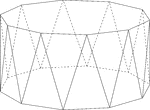### Decagonal Antiprism

Illustration of a decagonal antiprism. An antiprism is formed by having two parallel congruent bases…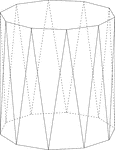### Decagonal Antiprism

Illustration of a decagonal antiprism. An antiprism is formed by having two parallel congruent bases…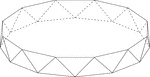### Dodecagonal Antiprism

Illustration of a dodecagonal antiprism. An antiprism is formed by having two parallel congruent bases…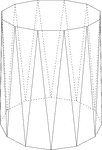### Dodecagonal Antiprism

Illustration of a dodecagonal antiprism. An antiprism is formed by having two parallel congruent bases…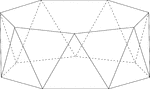### Heptagonal/Septagonal Antiprism

Illustration of a heptagonal, or sometimes known as a septagonal antiprism. An antiprism is formed by…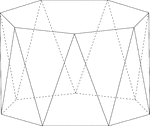### Heptagonal/Septagonal Antiprism

Illustration of a heptagonal, or sometimes known as a septagonal antiprism. An antiprism is formed by…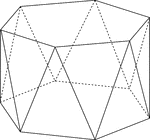### Hexagonal Antiprism

Illustration of a hexagonal antiprism. An antiprism is formed by having two parallel congruent bases…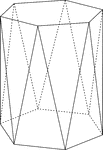### Hexagonal Antiprism

Illustration of a hexagonal antiprism. An antiprism is formed by having two parallel congruent bases…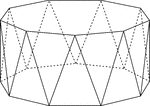### Nonagonal Antiprism

Illustration of a nonagonal antiprism. An antiprism is formed by having two parallel congruent bases…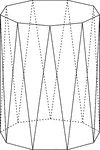### Nonagonal Antiprism

Illustration of a nonagonal antiprism. An antiprism is formed by having two parallel congruent bases…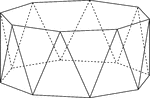### Octagonal Antiprism

Illustration of an octagonal antiprism. An antiprism is formed by having two parallel congruent bases…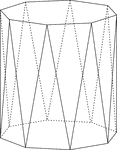### Octagonal Antiprism

Illustration of an octagonal antiprism. An antiprism is formed by having two parallel congruent bases…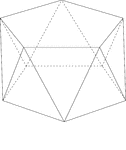### Pentagonal Antiprism

Illustration of a pentagonal antiprism. An antiprism is formed by having two parallel congruent bases…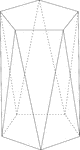### Pentagonal Antiprism

Illustration of a pentagonal antiprism. An antiprism is formed by having two parallel congruent bases…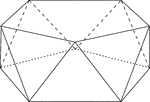### Pentagonal Antiprism

Illustration of a pentagonal antiprism. An antiprism is formed by having two parallel congruent bases…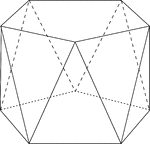### Pentagonal Antiprism

Illustration of a pentagonal antiprism. An antiprism is formed by having two parallel congruent bases…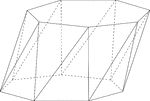### Skewed Heptagonal/Septagonal Antiprism

Illustration of a skewed (non-right) heptagonal, or sometimes known as a septagonal antiprism. An antiprism…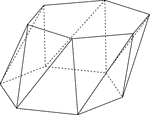### Skewed Hexagonal Antiprism

Illustration of a skewed (non-right) hexagonal antiprism. An antiprism is formed by having two parallel…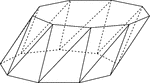### Skewed Nonagonal Antiprism

Illustration of a skewed (non-right) nonagonal antiprism. An antiprism is formed by having two parallel…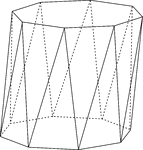### Skewed Octagonal Antiprism

Illustration of a skewed (non-right) octagonal antiprism. An antiprism is formed by having two parallel…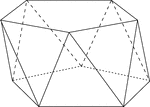### Skewed Pentagonal Antiprism

Illustration of a skewed (non-right) pentagonal antiprism. An antiprism is formed by having two parallel…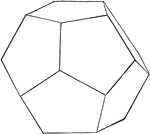### Regular Dodecahedron

Illustration of a regular dodecahedron. A regular dodecahedron is a polyhedron with twelve equal pentagonal…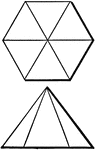### Drawing Lines 3

Top and side views of a hexagonal pyramid shaded with dimension lines.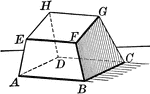### Hexahedron

Hexahedron - polyhedron with 6 faces.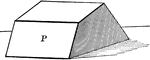### Hexahedron

Hexahedron - polyhedron with 6 faces.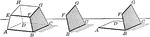### Hexahedron With Faces and Vertices

Hexahedron with faces and vertices shown.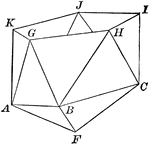### Part of an Icosahedron

Illustration of the bottom part of an icosahedron. The base consists of a regular pyramid, upon which…### Partial Construction of an Icosahedron

Illustration of the steps to a construction of an icosahedron. The base consists of a regular pyramid,…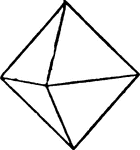### Octahedral Crystal

An illustration of an octahedral crystal. An octahedron is a polyhedron with eight faces. A regular…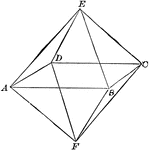### Regular Octahedron

Illustration of a regular octahedron. A polyhedron with eight equal faces.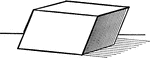### Parallelopiped

Illustration of a parallelopiped - a prism with a parallelogram as its base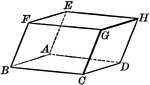### Parallelopiped

Illustration of a parallelopiped - a prism with a parallelogram as its base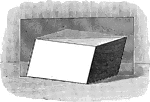### Parallelopiped with Plane Passing Through

Illustration of a parallelopiped with a plane passing through two diagonally opposite edges. The plane…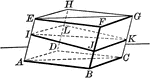### Parallelopiped with Plane Passing Through

Illustration of a parallelopiped with a plane passing through two diagonally opposite edges. The plane…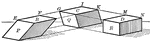### Parallelopiped Showing Volume

Illustration of a parallelopiped (a prism with a parallelogram as its base) used to demonstrate that…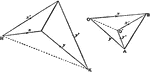### Polyhedron

Orthogonal projection of a closed plane-faced polyhedron.### Similar Polyhedrons

Two similar polyhedrons may be decomposed into the same number of tetrahedrons similar, each to each,…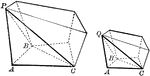### Two Similar Polyhedrons

Diagram used to prove the theorem: "Two similar polyhedrons may be decomposed into the same number of…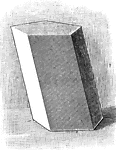### Prism

Illustration of a prism - a polyhedron of which two faces are equal polygons in parallel planes, and…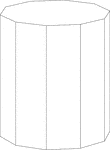### Decagonal Prism

Illustration of a right decagonal prism with regular decagons for bases and rectangular faces.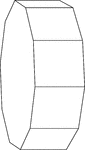### Decagonal Prism Resting On Its Side

Illustration of a right decagonal prism with regular decagons for bases and rectangular faces. The prism…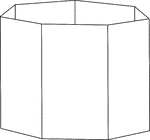### Heptagonal/Septagonal Prism

Illustration of a hollow right heptagonal/septagonal prism with regular heptagons/septagons for bases…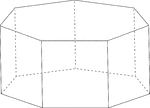### Heptagonal/Septagonal Prism

Illustration of a regular right heptagonal/septagonal prism with regular heptagons/septagons for bases…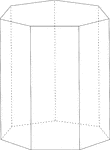### Heptagonal/Septagonal Prism

Illustration of a right heptagonal/septagonal prism with regular heptagons/septagons for bases and rectangular…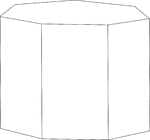### Heptagonal/Septagonal Prism

Illustration of a right heptagonal/septagonal prism with regular heptagons/septagons for bases and rectangular…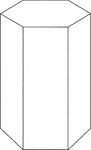### Hexagonal Prism

Illustration of a right hexagonal prism.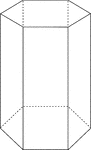### Hexagonal Prism

Illustration of a right hexagonal prism with hidden edges shown.### Hexagonal Prism

Illustration of a right hexagonal prism with a height less than the length of the edge of the hexagon.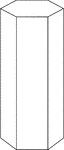### Hexagonal Prism

Illustration of a right hexagonal prism with a height greater than the length of the edge of the hexagon.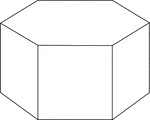### Hexagonal Prism

Illustration of a right hexagonal prism with hexagons for bases and square faces.### Hexagonal Prism

Illustration of a right hexagonal prism with hexagons for bases and square faces. The hidden edges are…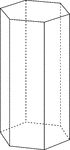### Hexagonal Prism

Illustration of a right hexagonal prism with hexagons for bases and rectangular faces. The height of…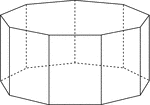### Nonagonal Prism

Illustration of a right nonagonal prism with regular nonagons for bases and rectangular faces. The hidden…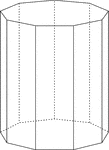### Nonagonal Prism

Illustration of a right nonagonal prism with regular nonagons for bases and rectangular faces. The height…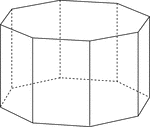### Octagonal Prism

Illustration of a right octagonal prism with regular octagons for bases and rectangular faces. The hidden…### Octagonal Prism

Illustration of a right octagonal prism with regular octagons for bases and rectangular faces. The height…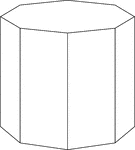### Octagonal Prism

Illustration of a right octagonal prism with octagons for bases and rectangular faces. The height of…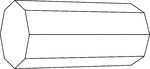### Octagonal Prism Resting On Side

Illustration of a right octagonal prism, with octagons for bases and rectangular faces, that is resting…